Reach Us+32-10-28-02-25
Yang-Mills Mass Gap Clay Institute Problem Solution | OMICS International
Journal of Physical Mathematics
All submissions of the EM system will be redirected to Online Manuscript Submission System. Authors are requested to submit articles directly to Online Manuscript Submission System of respective journal.

Yang-Mills Mass Gap Clay Institute Problem Solution

Cusack P*

Corresponding Author:
Cusack P
Independent Researcher
Tel: (506)214-3313
E-mail: [email protected]

Received date: February 08, 2016; Accepted date: April 29, 2016; Published date: May 05, 2016

Citation: Cusack P (2016) Yang-Mills Mass Gap Clay Institute Problem Solution. J Phys Math 7:171. doi:10.4172/2090-0902.1000171

Copyright: © 2016 Cusack P. This is an open-access article distributed under the terms of the Creative Commons Attribution License, which permits unrestricted use, distribution, and reproduction in any medium, provided the original author and source are credited.

Visit for more related articles at Journal of Physical Mathematics

Abstract

Here is the solution to the Yang-Mills Mass Gap Problem as described below. The Mass in the material universe is a balance between the resistance to mass formation (cuz=Pi-base e), squeezing Energy, and Gravity pulling it apart. There is an explanation as to why there are 116 sable elements in the material universe. The calculations are verified by predicting the size of the universe within experimental error.

Keywords

Quantum physics; Elementary particle theory; Yang-Mills theory

Introduction

The laws of quantum physics stand to the world of elementary particles in the way that Newton's laws of classical mechanics stand to the macroscopic world. Almost half a century ago, Yang and Mills introduced a remarkable new framework to describe elementary particles using structures that also occur in geometry. Quantum Yang- Mills theory is now the foundation of most of elementary particle theory, and its predictions have been tested at many experimental laboratories, but its mathematical foundation is still unclear. The successful use of Yang-Mills theory to describe the strong interactions of elementary particles depends on a subtle quantum mechanical property called the "mass gap": the quantum particles have positive masses, even though the classical waves travel at the speed of light. This property has been discovered by physicists from experiment and confirmed by computer simulations, but it still has not been understood from a theoretical point of view. Progress in establishing the existence of the Yang-Mills theory and a mass gap will require the introduction of fundamental new ideas both in physics and in mathematics.

Literature Search

As for a literature search on this topic, there are no Mathematical Physics models that I know about that assume a static universe in compression. All the theories purpose incorrectly that the universe is expanding. In order to understand the Mass Gap problem, we need to understand that there is a force on the exterior of the universe that is compressing the “dust” or “clay particles” together. Mass precipices. The only place you find this theory is in my 6 volume work entitled, Astrotheology, The Missing Link, and Cusack’s Model of the Universe [1,2].

Explanation

To begin, the Total Energy in the universe follows the e^-t, because the universe exist where the functiuon equals the derivative (y=y’=telescopic) function while the Kinetic Energy follows the Ln function. The difference is the Potential Energy stored in Mass, called Rm and is equal to cuz=Pi-e=0.4233 (Figure 1). In my work Astrotheology, The Missing Link, I’ve calculated that Gravity=Pi/ Ln 1.618. When t=Pi or the human frequency is 1/Pi=31.8 Hz, then humans perceive the Energy being squeezed into a Mass signal. Time is determined to follow the Golden Mean Equation. t^2-t-1=0, roots, t=- 0.618, 1.618. I call this the Energy parabola . Space is formed by the cross product of E and t is:

s=|E||t| sin θ

s=F

F=sin θ

We know in a harmonic system, the Cairnaut Second Order Linear Ordinary Differential Equation applies to the wave energy So,

∂E ^ 2 / ∂t − E = 0

I’ve shown G=E1

Gravity is that irreducible value that occurs when,

y=y’

y=E (Figure 2).

So the volume of this half cube is,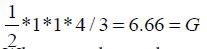When we subtract the weak nuclear force of of G=6.67, we get,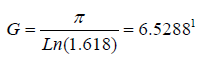Now the Resistance to Mass formation is bounded by the right triangle and determined by Pythagoras theorem.

(Lnπ ) ^ 2 + (e ^π ) ^ 2 = (Rm) ^ 2

Rm=π-e (Figure 3)

This illustrates that when space and time are equal, and when the force on the exterior of the universe in compression, equals sin theta, t6he universe we see at 31.8 Hz is tuned to human perception.

ρ=π/4-Re; Where ρ=density; Re=Reynold’s number,

Sin=ax+by+cz.

The force follows as in ewave; the space and time follow the plane.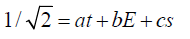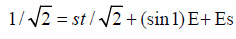Equation of the Universe

1/ sqrt2 = s / sqrt2*t2 + (sin1+ s)E

Knowing E=1/t,

t2+0.89207(1/t)-3/4=0

t3+c2-0.75=0

Let t=1

13+c2-0.75=0

C=1/2=R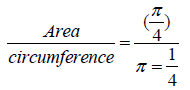4A=circumference

s=|E||t|cosθ

θ=60° Mohr-Coulomb Failure Plane

Due to the internal s structure of the Kaolinite, the universal material fails at 60 degrees.

Poisson’s Ratio

λ =ν *E / [(1+μ )(1− 2μ)]

m=0.27 this is Poisson’s Ratio for universal material

E=1/cuz

λ=1.0918

k=2π/λ

=5.7552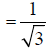f = A sin (ωt − kr ) + B cos (ωt − kr )

ω=1; 0.15=0.1666=600/3600

Where

A = −u0*a2 *cos(ka) + (ka)(sin(ka)] / (1+ (ka)2 )

u0=1

a=28.7

The universe exists where the Momentum, Force are coincide. The derivative is equal to the function. s=v=a

A=0.8475; s=v=a=sin1

And

B = −u0*a2sin(ka) − (ka)cos(ka) / [1+ (ka)2 = −0.6313 ~ G

F = sinθ = cosθ = P

F = −0.4272 − 0.2824

~ (π − 2a)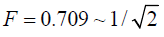(π − 2a) = sinθ = cosθ

θ=45°

So there are 8 cycles in a complete period of 2π.

1/81=0.12345679; 8 is missing. The mass figure crest over 1 at decimal 8 (Figure 4). The universe is where the cylindrical, polar, and Cartesian coordinate systems coincide. There is an inside and an outside to the known universe. An energy wave travels in the outer cylinder which reaches a climax at the inner universe ceiling. Actually, the force of this universal earthquake is such that the energy level is just right to cause space, and mass to precipitate. The force acting on the inner universe is sinθ .

E =1/ cuz, μ = 0.,27, ρ = 0.127, F =π − 2a

u / u0 = f (r / a) sin(ωt −φ)

u / u0 = f (a / a) sin(1− 2a / Pi)

u / u0 = f (1) sin (π /π − 2a /π)

=Sin1=s=v=a (Figure 5)

The periodic wave that travels in the outer core or cylinder of the tubular universe, reaches the right force (F=sin theta=8/3) at the outer edge of the ellipsoid thereby squeezing the Energy by the right amount to precipice mass and space and time.

V = 2π R2[(1−π / 4)r − R / 6]

Substituting, t=1.0337, 60°=1/6th circle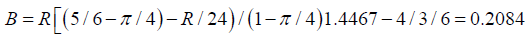Finally we achieve

σ=Eε

F(ε)=1/(π-e)

8/3(ε)=1/0.4233=0.8859=h=k

Where v=0.27 ωa/c=6

(1) a/4.3079=6.67a=28.7=r (Figure 6)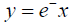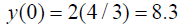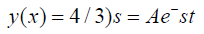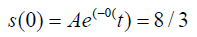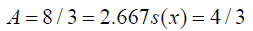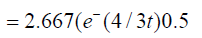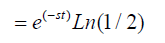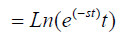= 0.5199 0.52

t-29.788°=30° if t=0.5199t/22LY=0.0236=1/0.4233=1/ cuz=E=youngs Modulus

And

t=0.5199t/66=0.0079

=1/(126.9)

=1/ρ

So there you have it v=0.27, E=1/0.4233, and ρ=0.127 (Figures 7-9)

To verify the calculations of the outer and inner universe, the dimension of the outer ring was determined to be within experimental error 

4/3+28.9+4/3=Diameter

(1/2)*(1/R2)=(1/2)*(1/c2)

R2=1/2(5.55)=0.9009=c2

C2=R

Area=πR2 = π(c2)circum.=2π*R=2π*c2

Area/Circumference=1/2

∂s / ∂t =| E | t | cosθ

θ=60°

1/2(1) (1) 1/2=1/4

4Area=circu. R=1/2

So,

C2=1/2c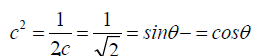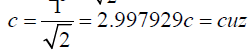The Universe is 13.34+0.289=13.629, within 13.73+/-0.12 range=13.61. This confirms my entire theory, My calculations agree with observation.

F=Ma

8.3=(Mproton+M electron)=(943+1.602)a=9.445a; a=2.823

2.823/cuz=6.668~G=6.67To produce Mass, some much Work goes into the Density and so much goes into the Spring or overcoming the charge resistance. I calculate it as follows:

Mp+Me-=943+1.602=944.6

Why are there 116 stable elements in the Periodic table whereas there should be 118?

944.6 x 2=1189.2

ΔF m=119.2/1189.2/0.4233 2809

ΔF=sin θ=2809-26.667=0.86441

arcsinθ (86441)=59.8157 degrees

sin 60°-sin 59.81 0.001613 ~ 1.618=t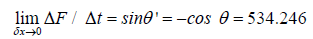θ=57°

60°- 57°=3°

sin 3°=0.05230.0523/2.667

=196(Infinity)

=196/2

=981

1/981

0.1019

N'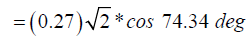1/N '=e

Δ F = {M p +M e −}* 2 / cuz

ΔF = {M p + Me −}*2 / {π −1/ N '}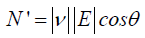2(4.486) / ΔF =π −1/ (0.27)cos 60 (Mass=4.486)

ρ=0.1272

Now

ΔF=2809

χ*ρ=ΔF

χ*=2.2-083χ(1/3)=1.3022

Continuing,

2M/ΔF=ET-1/Ev

((2*944.6)*2)=π-1/vcosθ

[2*(2M)-ρ]/[ΔF]=π

Or

4Fc2-ρ=Ft

F=1/cuz=E

Work Spring+Work Density=Work (Total)

4(t/cuz)c2-ρ=Ft

Or

Ρ=[Ft-Mc2]/4

Cusack's Mass Formation Equations

ρ=π/4-Re (Figure 10)

If we take a stress cube out of the cylindrical wall, we find that, when theta=60 degrees (Mohr coulomb Failure Plane), the sine wave force is enough to overcome the the resistance to mass Rm 2.667+4=6.67=G,

∂/L0=ε=σ/E

=4/(1/cuz)=1.6932

∂/L0=PL/AE

1.6392=(2.667)L3/AE

1.6392=2.667(4/3)πR2*(1/0.4233)

=2/π=dM/dt*freq

ε=freq*dM/dt

ε=σ/E=freq*dM/dt

Note

∫ε = Δ

2E *1/π (M) =σ

2(1/0.4233)*(1/π)M=1.52; M=1 Mass Gap

Finally,

Newton’s Gravitational Formula:

F<G M1M2/R^2

F< 2/3 M(1)/(1)^2

1.5F < M

1.5 (1)<M

1.5<M QED

Using Divergence, the Mass Gap can be found another way, as follows: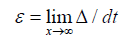Where

div∇=Scalar Field=Area of the circle with radius=1

1/τ=1/c area of the vector field where c=speed of light. This is a complete and closed system in Hilbert Space.

σ=E×ε=(π−e)×ε=(0.4233)(1−sin1)

π−e=cuz=Resistance of Energy to Mass formation.

ε=δL/L=Moment=1-sin 1=0.1585=0.8415=sin 1

And,

Area=circumference of a circle,

y=y'

πR2=2πR

R=1

Why is the sin (1 rad) required?

S=|E||t|sintheta

E=1, t=1

s=sinθ

Dot Product=Cross Product

|E||t|cosθ=|E||t|sinθ

−sinθ=cosθsinces=v=a=s=s’=s’’

Θ=45degrees

But s=t=1

Sin θ=sin t=sin1=0.8415

Lorentz Transform=√[1+v2/c2]

=sqrt[1+0.84152/2.99792]=0.8481

So, continuing

div∇=1/∇=1/δτtau∗∇2/2*E*Ïµ

div∇∗δτ=π2/2(π−e)(1−sin1)

δτ=1/c

So,

div∇=c/2∗∇2*σ

div∇/(∇2*σ)=1.5=Mass gap

A2/(π2*F)=1.5

π2/π2/F=1.5

1/F=Mass Gap

The Super force is F=sin theta=sin t where t=1. (AstroTheology, Cusack’s Universe)

1/sin 1 rad=118.8 Mass of the last element 118 in the periodic table.

The equation of the universe is,

∫ba1/n!=E=ex

There are 118 chemical elements. So,

Ln (1/n!)=Ln (1/118!)=4.482=Mass

E=Mc2=4.482(2.997929)^2=0.402=Reynold’s Number.

0.402/c=0.402/2.9979=0.1341

Conjugate=1-0.1341=0.866=sin 60 degrees=critical angle of repose for kaolinite Universe.

QED (Figure 11)

And, the Dirac delta function,

delta={1/c for |x|=|t|=c/2=3/2=1.5} (Actually, 1.499 as above)

Conclusion

The mass gap exists because as we've seen in these last few pages again, the gravity would pull apart any atoms above 6.67 or n=4+tau=2.667; and it takes f=2.667 at 60 degrees to compress energy into the form mass. The resistance to massisrm=cuz=pi-e.

References

Select your language of interest to view the total content in your interested language

Article Usage

• Total views: 10022
• [From(publication date):
June-2016 - Oct 21, 2019]
• Breakdown by view type
• HTML page views : 9839Can't read the image? click here to refresh